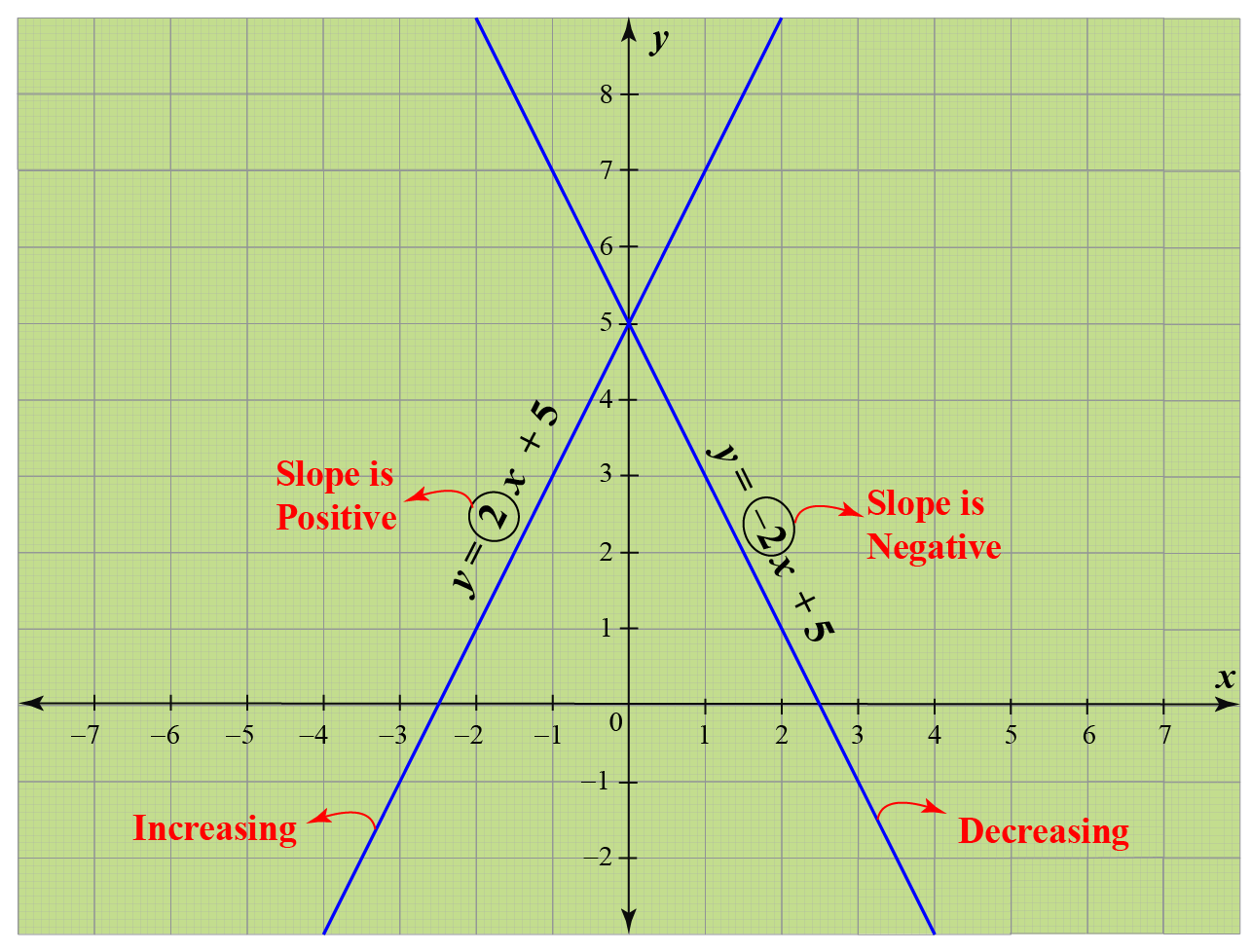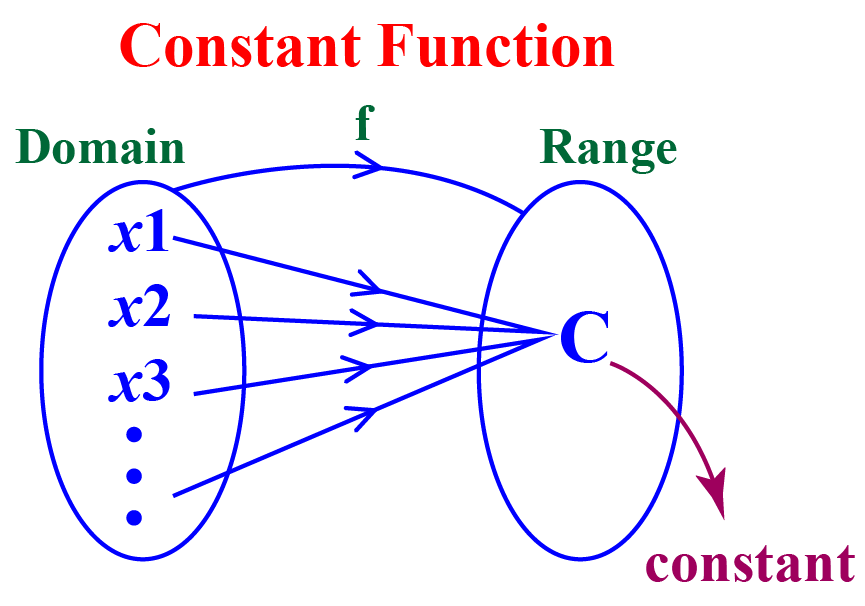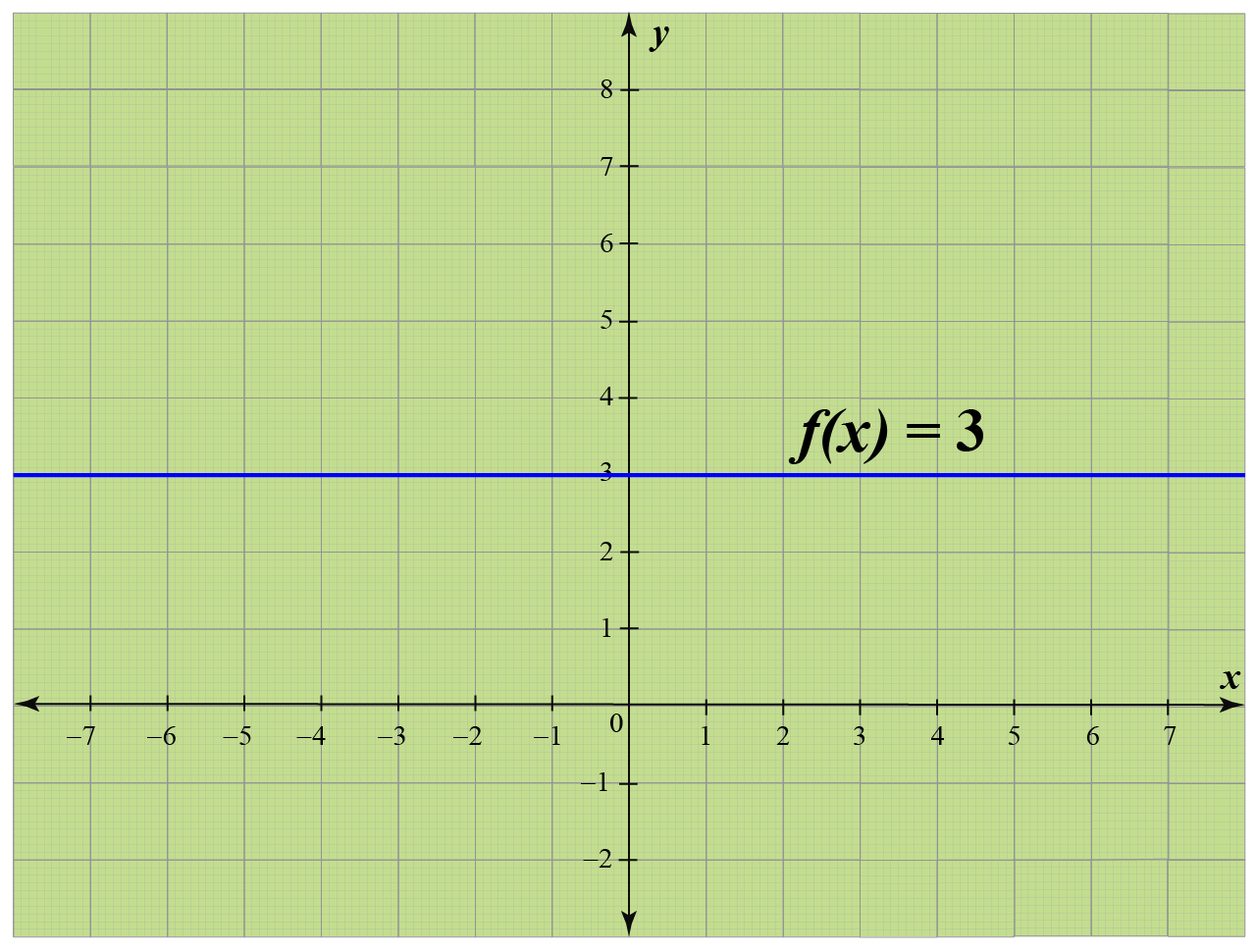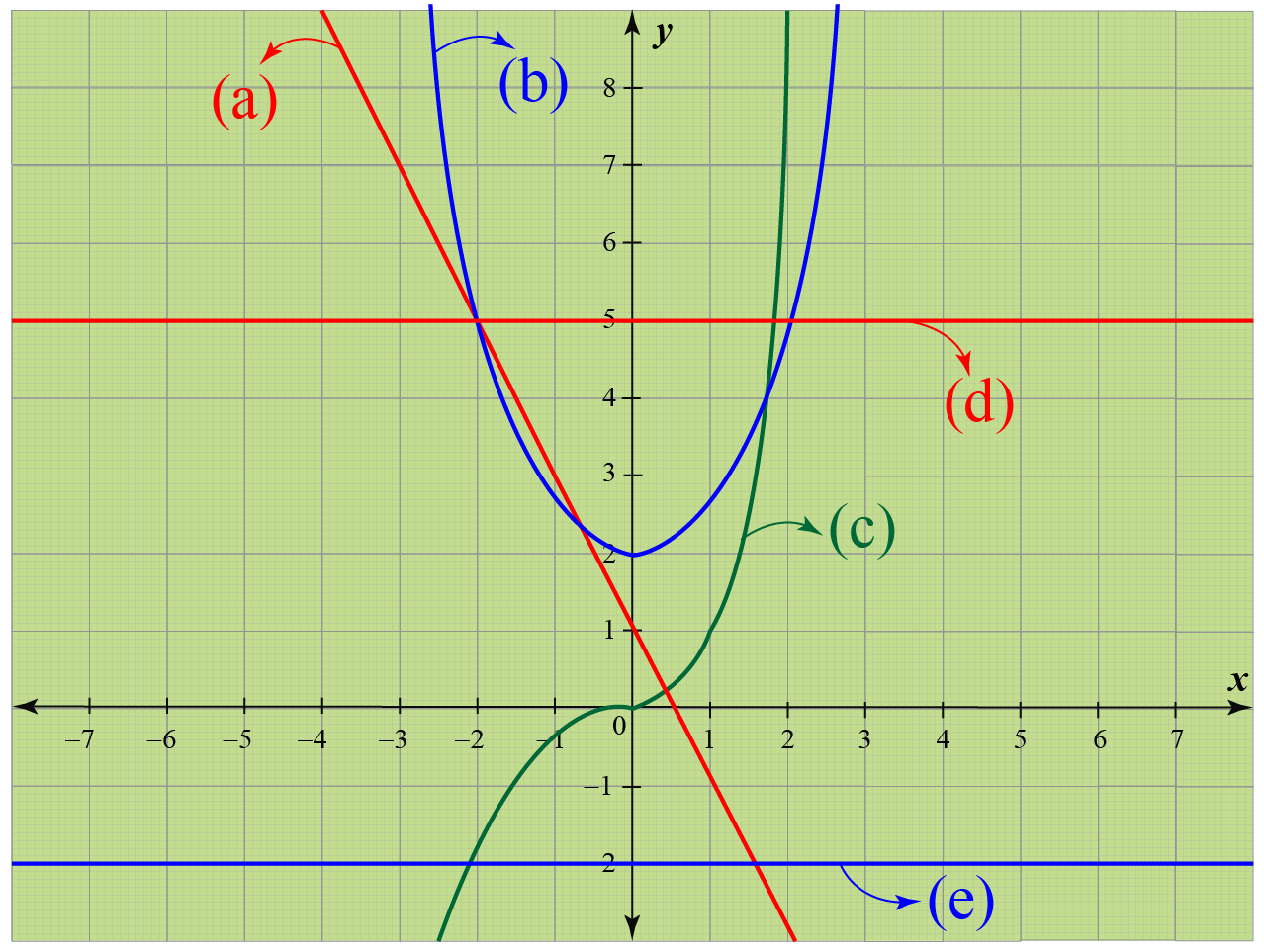# Constant Functions

Constant Functions

The functions can be increasing, decreasing, and constant functions.

We know that a function is

• Increasing on its domain if it is rising continuously from left to right.
• Decreasing on its domain if it is falling continuously from left to right.

In particular, a function representing a straight line increases when its slope is positive and decreases when its slope is negative.But is there any function representing a straight line that is neither increasing nor decreasing?

Yes, and that kind of functions are called constant functions.

Let's learn more about constant functions here.

## Lesson Plan

 1 What Do You Mean by a Constant Function? 2 Tips and Tricks on Constant Functions 3 Solved Examples on Constant Functions 4 Interactive Questions on Constant Functions 5 Challenging Questions on Constant Functions

## What Do You Mean by a Constant Function?

A constant function is a linear function whose range contains only one element irrespective of the number of elements of the domain.Consider the function $f(x)=3$

There is no variable in the definition (on the right side).

This means that it will always generate an output equal to 3, no matter what input value we give to it.

Hence, this particular function is a constant function.

Thus, a constant function is of the form

 $$f(x)=k$$, where $$k$$ is a constant.

## Graphs of Constant Functions

From the above explanation, a constant function refers to a real-valued function with no variable in its definition.

Let us consider the constant function $$f(x)=3$$ where $$f: R \rightarrow R$$.

This means that it will always generate an output equal to 3, no matter what input value we give to it.

So some points on its graph can be (-1, 3), (2, 3), (4, 3), etc.

Let us just plot all such points, join them, and see what we get.So the graph of $$f(x) =3$$ is a horizontal line as the y-coordinates of all points are the same (as 3)

Hence,

 The graphs of all constant functions are horizontal lines.

## Characteristics of a Constant Function

### Slope of a Constant Function

We know that the constant function is of the form $$f(x)=k$$ (or) $$y=k$$.

This can be written as $$y=0x+k$$.

Comparing this with the slope-intercept form $$y=mx+b$$, we get its slope to be $$m=0$$.Thus,

 The slope of a constant function is 0

### Domain and Range of a Constant Function

Since the constant function is defined for all real values of $$x$$, its domain is the set of all real numbers, $$\mathbb{R}$$.

Since a constant function $$f(x)=k$$ leads to only one output, which is $$k$$, its range is the set with just one element $$k$$.

 Domain = $$\mathbb{R}$$ Range = $$\{k\}$$

Apart from these, some other characteristics of constant functions are:

• The constant functions are continuous as they represent horizontal lines that extend continuously on both sides without any break.
• The constant functions are measurable because if $$f:A \rightarrow \{k\}$$ is a constant function, then inverse image set of $$f$$ is either $$\phi$$ or $$A$$.Tips and Tricks
1. The graph of a constant function can never be a curve.
2. The graph of a constant function is always a horizontal line.
3. An algebraic function is a constant function if there is no variable in its definition.

## Constant Functions in Real World

Here are some examples of constant function in the real world.

• The price of any item at a departmental store is $3. • In a book sale, the price of any book is$10.
• A bag worth $30 is free for all the purchases that go beyond$300.
• The maximum marks that can be obtained in an examination.

## Solved Examples

 Example 1

Can we help Jim identify the constant function(s) among the following?

a) $$y = -2x+3$$
b) $$\pi -y=0$$
c) $$x^2+y^2=1$$
d) $$y-2=0$$
e) $$y=x$$

Solution

We first solve each equation for $$y$$. Then the given equations become:

a) $$y = -2x+3$$
b) $$y=\pi$$
c) $$y= \sqrt{1-x^2}$$
d) $$y=2$$
e) $$y=x$$

Among these, the ones with just the constant on the right-hand side part are $$y=\pi$$ and $$y=2$$.

 $$\therefore$$ The constant functions are b) and d).
 Example 2

Which of the following would represent the constant function(s)?Solution

We know that the graph of a constant function is always a horizontal line.

Among the given graphs, only (d) and (e) are the horizontal lines.

 $$\therefore$$ The constant functions are  (d) and (e).

## Interactive Questions

Here are a few activities for you to practice.

Select/type your answer and click the "Check Answer" button to see the result.Challenging Questions
1. Is the graph of a vertical line a constant function?
Hint: Think whether it is a function. You can apply the vertical line test.
2. Plot the graph of the following constant function:
$f(x)=\left\{\begin{array}{ll} 1, & x \in[-1,2] \\ 2, & x \in(2,3] \end{array}\right.$

## Let's Summarize

The mini-lesson targeted the fascinating concept of Constant Functions. The math journey around Constant Functions starts with what a student already knows, and goes on to creatively crafting a fresh concept in the young minds. Done in a way that not only it is relatable and easy to grasp, but also will stay with them forever. Here lies the magic with Cuemath.

## About Cuemath

At Cuemath, our team of math experts is dedicated to making learning fun for our favorite readers, the students!

Through an interactive and engaging learning-teaching-learning approach, the teachers explore all angles of a topic.

Be it worksheets, online classes, doubt sessions, or any other form of relation, it’s the logical thinking and smart learning approach that we, at Cuemath, believe in.

## FAQs on Constant Functions

### 1. What is the equation of a constant function?

The equation of a constant function is of the form f(x) = k, where 'k' is a constant.

### 2. How do you know if a function is constant?

If the definition of a function has no variable in it, then it is a constant function.

For example, f(x) = 5 is a constant function.

### 3. Is a constant function linear?

The graphs of both linear and constant functions are straight lines.

So a constant function is linear.

In particular, it is a horizontal line always.

### 4. Can a constant function be onto?

Yes, a constant function f(x) = k can be onto when its codomain is as same as its range (which is {k}).

### 5. What is the degree of constant function?

The degree of a constant function is zero as a constant k can be written as kx0.

### 6. Is constant function surjective?

A constant function is NOT surjective as it is NOT one-one.

### 7. What is constant function and identity function?

A constant function is of the form f(x) = k, where 'k' is a constant.

An identity function is of the form f(x) = x.

### 8. Can a constant function be 1-1?

A constant function is one-one only when its domain has exactly one element.

### 9. What is the derivative of a constant function?

The derivative of a constant function is always zero.

### 10. Is a constant function a polynomial function?

Yes, every constant function is a polynomial function.

Download Functions Worksheets
Functions
grade 10 | Answers Set 1
Functions
grade 10 | Questions Set 2
Functions
grade 10 | Answers Set 2
Functions
grade 10 | Questions Set 1
More Important Topics
Numbers
Algebra
Geometry
Measurement
Money
Data
Trigonometry
Calculus